# Nodal Analysis - KCL and Matlab

A nodal analysis can be performed by examining each node in a circuit. The goal is to find out what the voltages are in each node with respect to our reference node. We need to know the currents flowing in the circuit and the resistances between each nodes. This is just an application of the Ohm's Law.

Kirchhoff’s current law (KCL) states that for any electrical circuit, the algebraic sum of all the currents at any node in the circuit is zero.

In this type of analysis, if there are n nodes in a circuit, and we select a reference node (node 0), the other nodes can be numbered from V1 through Vn-1.

With one node selected as node 0 (reference), there will be n-1 independent equations. If we assume that the admittance between nodes i and j is given as Yij, we can write the following equations including all of the nodes in the circuit: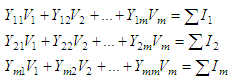where:

m = n - 1
V1, V2,..., Vm are voltages from all the nodes with respect to node 0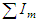is the algebraic sum of current sources at node m.

The above system of equations can be expressed in matrix form as:

Y V = I

And naturally, its solution is:

V = Y -1I, which in Matlab can be easily solved using the backslash operator (\), like this:

V = Y\I

The following example illustrates the use of Matlab for solving nodal voltages of electrical circuits.

### Nodal Analysis - find the voltages in a circuit

For the circuit shown below, resistors are in ohms and current sources are in amps. We’re asked to find the nodal voltages V1, V2, and V3.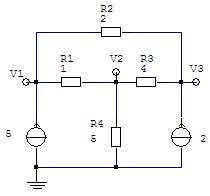Using KCL, and forming our matrices Y and I, let’s see...

For node V1 we have,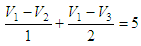and the first row of our Y matrix is going to be the coefficients for the voltages. This means that we can form our matrix in Matlab like this

Y(1,:) = [(1/1 + 1/2)  -1/1  -1/2];
and naturally I(1) = 5;

At node V2,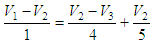the second row of our Y matrix is going to be the new set of coefficients for the voltages. Letting Matlab work out the operations, we express

Y(2,:) = [1/1  (-1/1 - 1/4 - 1/5)  1/4];
and I(2) = 0;

Finally, at node V3 we have,thus, our third row of the Y matrix is

Y(3,:) = [-1/2 -1/4 (1/2 + 1/4)];
and I(3) = 2;

Summarizing our findings, we fill-in our matrices Y and I, like this:

Y = 1.5  -1.00   -0.50
1.0  -1.45    0.25
-0.5  -0.25    0.75

I =  5
0
2

The solution is easy using Matlab: just type V = Y\I. The answer is matrix V, with three rows (our vector of unknowns contains three variables in a column: V1, V2 and V3)

V = 40.4286
35.0000
41.2857

An analysis with more components

From 'Nodal Analysis' to home